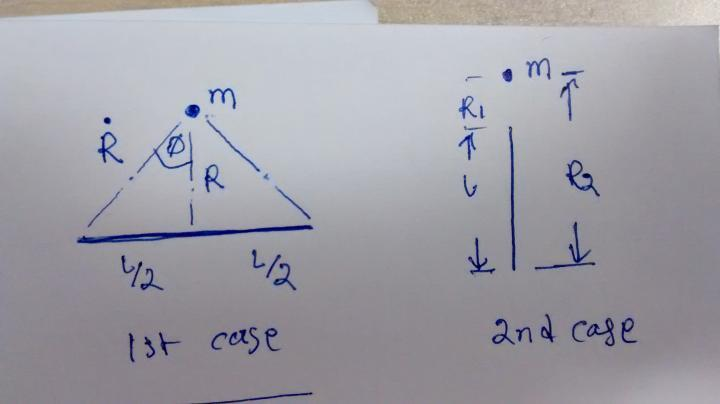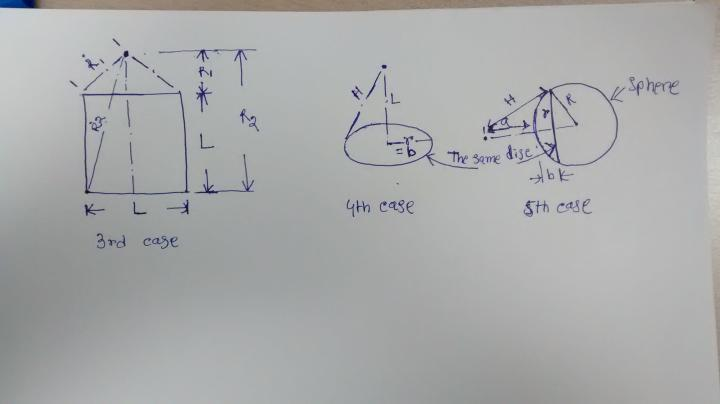igeazle portable/travel desk
It can be used in a variety of settings; its applications are endless.

FEATURED ARTICLE

## Investigating gravitational force by a large body on a small body

• Posted by AMITAVA
• August 22, 2014 3:05 PM EDT
• 1 comment
• 3,798 viewsFirst I will start with a straight strip with negligible thickness and depth. It has length L and mass per unit length M. The other object has mass "m" and has negligible dimension. The other object lies at a distance R from the strip on a line perpendicular to the strip and going through the mid of the strip. Now I want to study the nature of gravitational force by the strip on the object "m". Let us consider an infinitesimal length dx on this strip at a distance x from mid point. The gravitational pull of this infinitesimal portion on the mass “m" will have two components. One is straight towards the strip and another is perpendicular to it. The second force gets cancelled out if we consider both sides of the mid point. Only the first one will add up. Now writing the equation here for the force which is directed towards the strip.

(GmMdx/(R²+ x²))(R/√(R²+ x²)). Now integrating it over the half length (L/2) we get

∫(GmMdx/(R²+ x²))(R/√(R²+ x²)) = (GmMR)∫dx/(R²+ x²)3/2
Considering x/R = tanθ, we get dx = Rdθ/cos2θ,
substituting and rearranging

(GmMR)∫dx/(R²+ x²)3/2 = (GmMR)(1/R²)∫cosθdθ = (GmMR)(1/R²)sinθ =(GmM)(1/R)sinΦ,
considering θ changes from 0 to Φ over L/2, Now multiply and divide by L/2, we get Gm(Ṁ/2)/(RṘ), Where Ṁ = M*L And (L/2)/Ṙ= sinΦ, considering both sides of the mid point it becomes GmṀ/(RṘ). Now comparing with normal equation GmṀ/R², we can see GmṀ/R² > GmṀ/(RṘ), as Ṙ > R.

2nd case - Now the second mass "m" lies on the line of the strip but at some distance from the strip. In this case the integration is from R1 (nearest to "m") to R2 (farthest from "m") and very straightforward.

∫GmMdx/x2 = -∫(GmM*(1/x) = ∫(GmM/(1/R1 - 1/R2)= GmM(R2 - R1)/(R1R2)= GmṀ/(R1R2)
and GmṀ/(R1R2) > GmṀ/R2, where R = (R1 + R2)/23rd case - using the result from first case. now suppose the negligible thickness is dR (towards the direction of R). Now integrating GmṀ{dR/(RṘ)} over length L will give total force acting by the bigger object with area L*L on the smaller object.

∫GmṀ{dR/(RṘ)} = ∫GmṀ{dR/(R√(R2 +(L/2)2)} as Ṙ = √(R2 +(L/2)2)
dṘ=RdR/Ṙ, the integration becomes GmṀ∫{dṘ/(Ṙ2 -(L/2)2)}
or, (GmṀ/L)∫{(1/(Ṙ - L/2) - (1/(Ṙ + L/2)}dṘ
or, (GmṀ/L){ln(Ṙ - L/2) - ln(Ṙ + L/2)}
or, (GmṀ/L)ln{(Ṙ - L/2)/(Ṙ + L/2)}, integrating Ṙ from Ṙ1 to Ṙ2, we get
(GmṀ/L)ln[{(Ṙ2 - L/2)(Ṙ1 + L/2)}/{(Ṙ2 + L/2)(Ṙ1 - L/2)}]

or (GmḾ/L2)ln[{(Ṙ2 - L/2)(Ṙ1 + L/2)}/{(Ṙ2 + L/2)(Ṙ1 - L/2)}], where Ḿ = ṀL, and Ṙ2 = √(R22 +(L/2)2), Ṙ1 = √(R12 +(L/2)2) and R2 - R1 = L

or, (GmḾ/L2)ln[{(1 - L/(2Ṙ2)(1 + L/(2Ṙ1))}/{(1 + L/(2Ṙ2))(1 - L/(2Ṙ1))}] or arranging
(GmḾ/L2)(ln{(1 - L/(2Ṙ2)/(1 + L/(2Ṙ2)} - ln{(1 - L/(2Ṙ1)/(1 + L/(2Ṙ1)} now expanding both the logs and neglecting higher terms we get.
(GmḾ/L2)[ {-2(L/2Ṙ2)+ ------} + {2(L/2Ṙ1)+ ------} = (GmḾ/L2){L/Ṙ1 - L/Ṙ2 + ------} > (GmḾ/L){1/Ṙ1 - 1/Ṙ2} = {GmḾ/(Ṙ12)}{(Ṙ2- Ṙ1)/L}
Now it can be shown that {GmḾ/(Ṙ12)}{(Ṙ2- Ṙ1)/L} > GmḾ/R2, where R = (R1 +L/2) and Ḿ is the total mass of the object with area LL.

4th case- (Please see the second photo) Now consider a ring (whose cross section looks like a rectangle, not like a circle). Whose radius is "r" and density is as usual "M". Now the small mass "m" lies on the axis of the ring with distance "L" from the center of the ring. Thickness of the ring towards the direction of ring is dr (infinitesimally small) and the other thickness is dz (which I will not mention immediately, but assume it is there). Now the portion rdθdr will exert force GmMrdθdr/(L2 + r2), but towards the direction of the axis it will be
{GmMrdθdr/(L2 + r2)}(L/√(L2 + r2)), integrating it we get {GmM2Πrdr/(L2 + r2)}(L/√(L2 + r2)),again integrating it wrt r, from r = 0 to r = b we get GmM2ΠL(1/L - 1/H), where we assumed √(L2 + r2) = h (the distance between the portion rdθdr and the mass "m"), which will be L at r = 0 and H at r = b.

Finally we got the attraction force between the disc with radius "b" and mass "m" which is GmM2ΠL(1/L - 1/H).

5th case- Now consider the disc is part of a sphere of radius "R" and the thickness of the disc is dz (=dL) which I mentioned earlier. Now "L" (as well as "H") is variable and "L" has two parts, one is "a" which is outside the sphere and thus constant and another is "b" inside the sphere, so "b" is variable depending on the position of disc from the mass "m". Now if we integrate, we get
∫GmM2Π(1 - L/H)dL= ∫GmM2Π(1 - (a +b)/√((a+b)2 + r2)db
noting that r= √(R2 - (R-b)2), we get

∫GmM2Π(1 - (a + b)/√(2(a+R)b + a2)db, using earlier method we finally get the integration in the form

∫GmM2Π(db - (a/(a+R))dH - (1/(2(a+R)2)) (H2-a2)dh) where H changes from a to a + 2R, b changes from 0 to 2R. Now performing the integration and putting the initial and final values of "b" and "H" we get
GmM2Π{(2R3 - (4/3)R3)/(a+R)2} or Gm(M4ΠR3/3)/(a + R)2 or  {GmḾ/(a + R)2}, which is exactly what we assume that all the mass is concentrated at the center. This equation is valid only for a ≥0.

Okay now the "m" what we assumed as a point mass (negligible dimension)so far, also becomes a sphere of appreciable dimension, how do we calculate the resultant force now? Surprisingly we do not need to calculate anymore. By the property of sphere we already saw that for a sphere (of any radius) we can assume all the mass is concentrated at the center, so for a concentrated mass we can assume that the mass is uniformly distributed over a sphere (of any radius). The equation will remain same!

Let us take the disc again (4th case) and now consider it a part of cylinder. Now integrate it as usual

∫GmM2Π(1 - L/H)dl = GmM2Π{(L2- L1) - (H2- H1)} where L and H have the same meaning earlier. Now rearranging it

GmM2Π{(L2- H2) + (H1- L1)} and letting L2 →∞ so also H2 →∞ , we get GmM2Π(H1- L1), which is the upper bound! that means no matter how much we increase the size of the cylinder by increasing its length (only) it can not be more than (or equal to) GmM2Π(H1- L1). where H1 and L1 both are finite and L1 is the distance between the disc and the mass "m"

Now in the original case GmM2Π{(L2- L1) - (H2- H1)} and letting R (the radius of the cylinder) →∞ that means H →∞ we get GmM2Π(L2- L1)!!, where L2- L1 is the length of the cylinder (which has now become a disc because of shape).

This is outright spooky to me as this gravitational force "GmM2Π(L2- L1)" (which is finite and constant, depends on M (density), m, and (L2-L1) )does not depend on the distance between the infinite disc (with finite thickness) and the mass "m".

Note: the multipication symbol is not used to make it more legible.